# Social Media, UF Acceptances, 2019 COLLEGE FAIR, Scholarships & More!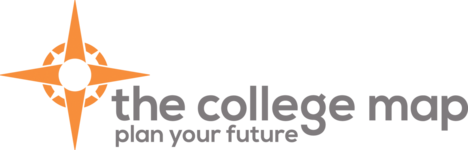# Hello, The College Map!

## Colleges Acceptances!

 /* styles */ Please be sure you continue to check your portals regarding acceptances for summer or fall admissions. There are next steps to be working on in the process: enrollment deposits (by May 1st), housing decisions and deposits, and financial aid award letters.
 table div table+table+table+table+table+table div table{width:100%;padding:0}table div table+table+table+table+table+table div table img{width:96.23%;padding:0;float:none}table div table+table+table+table+table+table div table td{width:100%;padding:0 1.88% 18px}/* styles */# Very proud of our hard working seniors!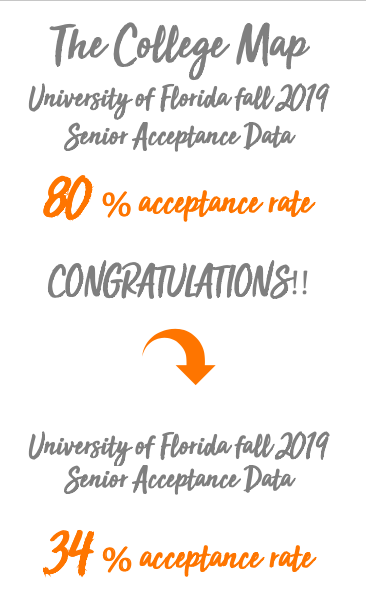table div table+table+table+table+table+table+table+table+table+table div table{width:100%;padding:0}table div table+table+table+table+table+table+table+table+table+table div table img{width:96.23%;padding:0;float:none}table div table+table+table+table+table+table+table+table+table+table div table td{width:100%;padding:0 1.88% 18px}/* styles */## 2019 TCM College Fair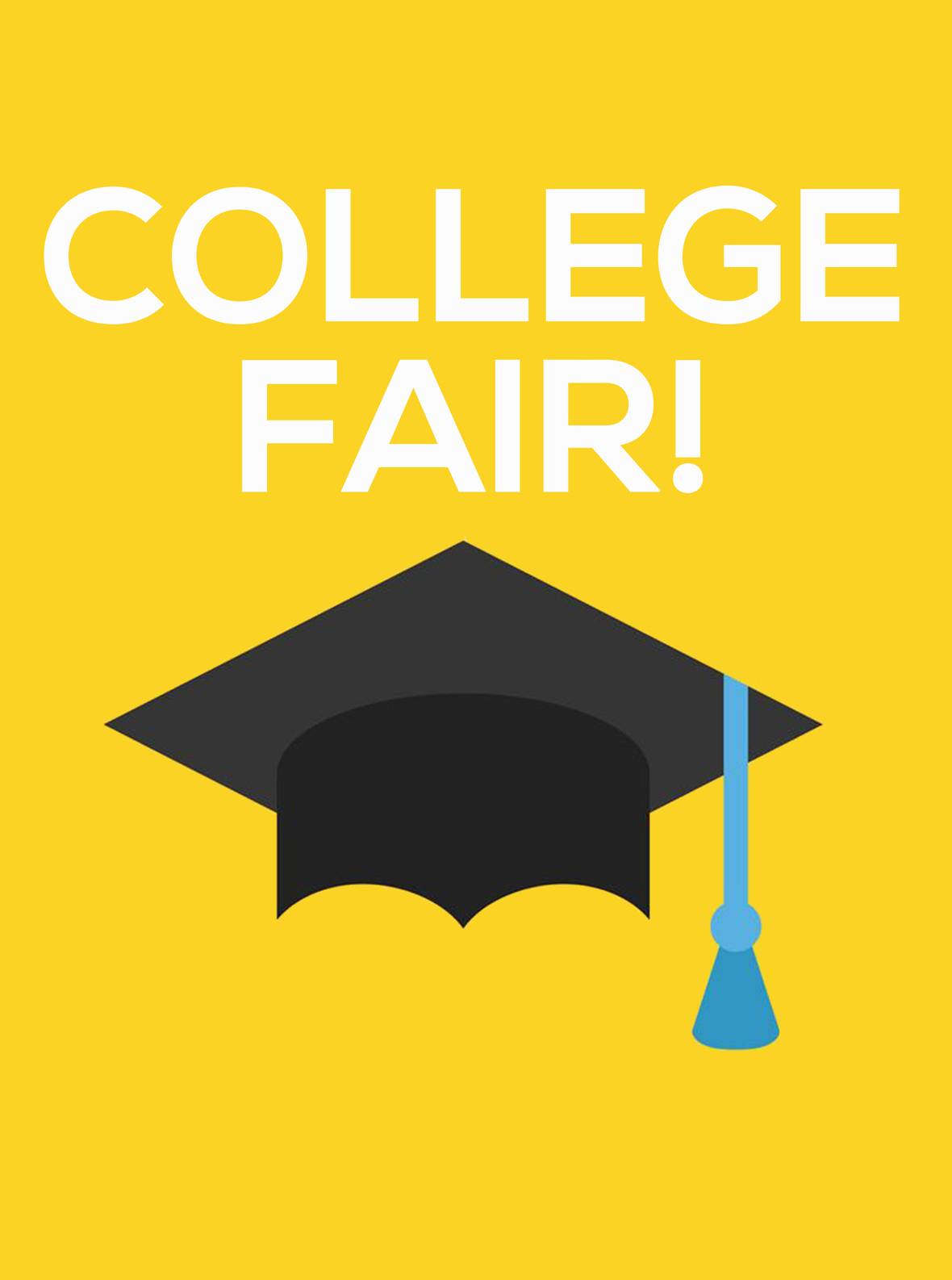# College Fair

February 22 from 3-5pm at The College Map

We're excited to announce our upcoming college fair!

Meet reps from:
Florida Atlantic University
Florida Gulf Coast University
Florida Institute of Technology
Florida State University
Johnson and Wales University
Ohio State University
Rollins College
Stetson University
University of Alabama
University of Central Florida
University of Florida
University of North Florida
University of Maryland
University of South Florida
University of West Florida!

Click HERE to register

 table div table+table+table+table+table+table+table+table+table+table+table+table+table div table{width:100%;padding:0}table div table+table+table+table+table+table+table+table+table+table+table+table+table div table img{width:96.23%;padding:0;float:none}table div table+table+table+table+table+table+table+table+table+table+table+table+table div table td{width:100%;padding:0 1.88% 18px}/* styles */## High School Classes Colleges Look For/* styles */ This is the time of year students start choosing their classes for next year. Please remember the courses you take now MATTER! Make sure to connect with your College Map Counselor regarding your schedule for next year. "To ready yourself for college-level work, enroll in challenging high school courses, such as honors classes, AP courses or IB-program courses. You may even be able to take college courses at your high school or a local college." Click here for the full article.
 table div table+table+table+table+table+table+table+table+table+table+table+table+table+table+table+table+table div table{width:100%;padding:0}table div table+table+table+table+table+table+table+table+table+table+table+table+table+table+table+table+table div table img{width:96.23%;padding:0;float:none}table div table+table+table+table+table+table+table+table+table+table+table+table+table+table+table+table+table div table td{width:100%;padding:0 1.88% 18px}/* styles */## Have You Googled Yourself Lately?/* styles */ You may think that picture your friend tagged you in is no big deal, but what if a prospective college admissions officer saw it? Would they notice anything that could hurt your chances of being placed in the admit pile? It seems every year there is a story about social media coming into play with admissions. We encourage all of our students to always do a social media audit and make sure their privacy settings are turned on and a simple Google search on their name doesn't bring up anything we wouldn't want a college to find. - CollegePrep360
 table div table+table+table+table+table+table+table+table+table+table+table+table+table+table+table+table+table+table+table+table+table div table{width:100%;padding:0}table div table+table+table+table+table+table+table+table+table+table+table+table+table+table+table+table+table+table+table+table+table div table img{width:96.23%;padding:0;float:none}table div table+table+table+table+table+table+table+table+table+table+table+table+table+table+table+table+table+table+table+table+table div table td{width:100%;padding:0 1.88% 18px}/* styles */## Rising Junior Diagnostic Testing Dates

 /* styles */ Sophomores enrolled in our test prep program are expected to pick one date to complete the ACT/SAT diagnostic to determine their strength test. Please email Mrs. Mitchell (juliemitchell@thecollegemap.com) with your date preference. Saturday, May 18th 1:00 p.m.-5:00 p.m. Sunday, May 26th noon-4:00 p.m. Monday, June 3rd noon-4:00 p.m. Tuesday, June 4th noon-4:00 p.m. Wednesday, June 5th noon-4:00 p.m. Thursday, June 6th noon-4:00 p.m. Monday, June 10th 10:00-2:00 p.m. Tuesday, June 11th 10:00-2:00 p.m. Wednesday, June 12th 10:00-2:00 p.m. Thursday, June 13th 10:00-2:00 p.m. Friday, June 14th 10:00-2:00 p.m.
 table div table+table+table+table+table+table+table+table+table+table+table+table+table+table+table+table+table+table+table+table+table+table+table+table div table{width:100%;padding:0}table div table+table+table+table+table+table+table+table+table+table+table+table+table+table+table+table+table+table+table+table+table+table+table+table div table img{width:96.23%;padding:0;float:none}table div table+table+table+table+table+table+table+table+table+table+table+table+table+table+table+table+table+table+table+table+table+table+table+table div table td{width:100%;padding:0 1.88% 18px}/* styles */## 2019 Test Prep CalendarsTest prep students, when you are registering for live classes utilizing our Acuity online scheduler, please make sure that you are signing up for the correct class based on your strength test. You should be signing up for either ACT or SAT classes, not the other and not both. This confusion is causing classes to fill up in error. Also, please do not sign up more than once for each individual class as this is also causing classes to fill up in error. We truly appreciate your CAREFUL attention to this matter.

Please sign up at our Acuity Scheduler website!
See below for our new 2019 calendars.

Please register for the ACT at act.org OR SAT at collegeboard.org. Please note there are early registration deadline dates. You can register after the deadline date but a late fee will be required.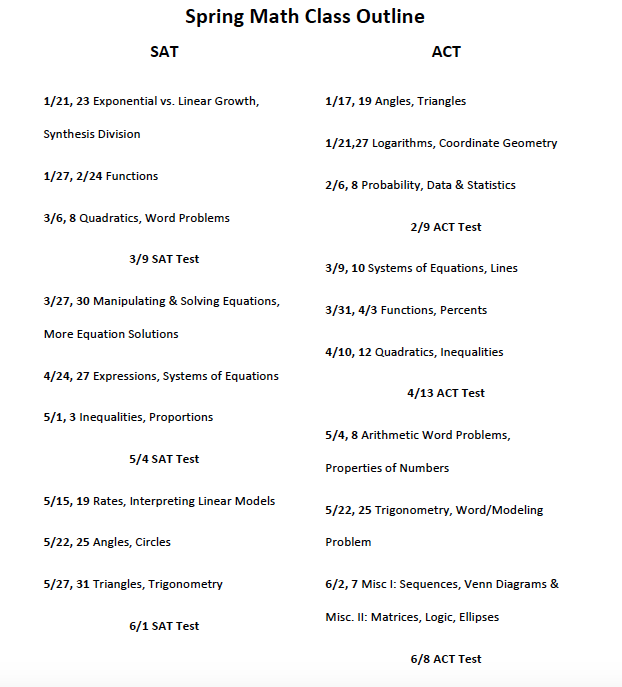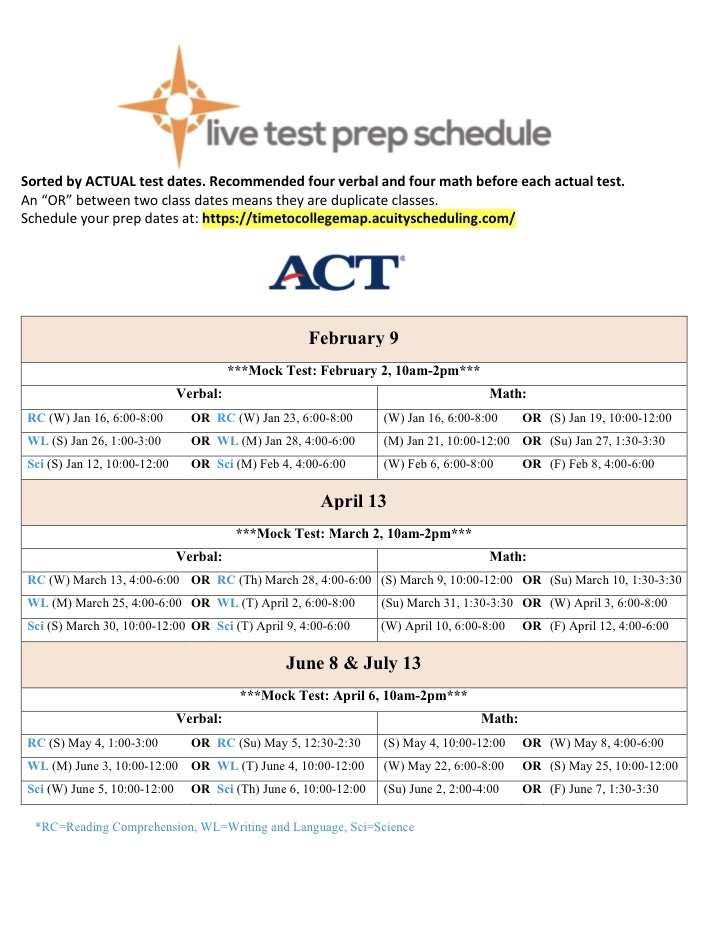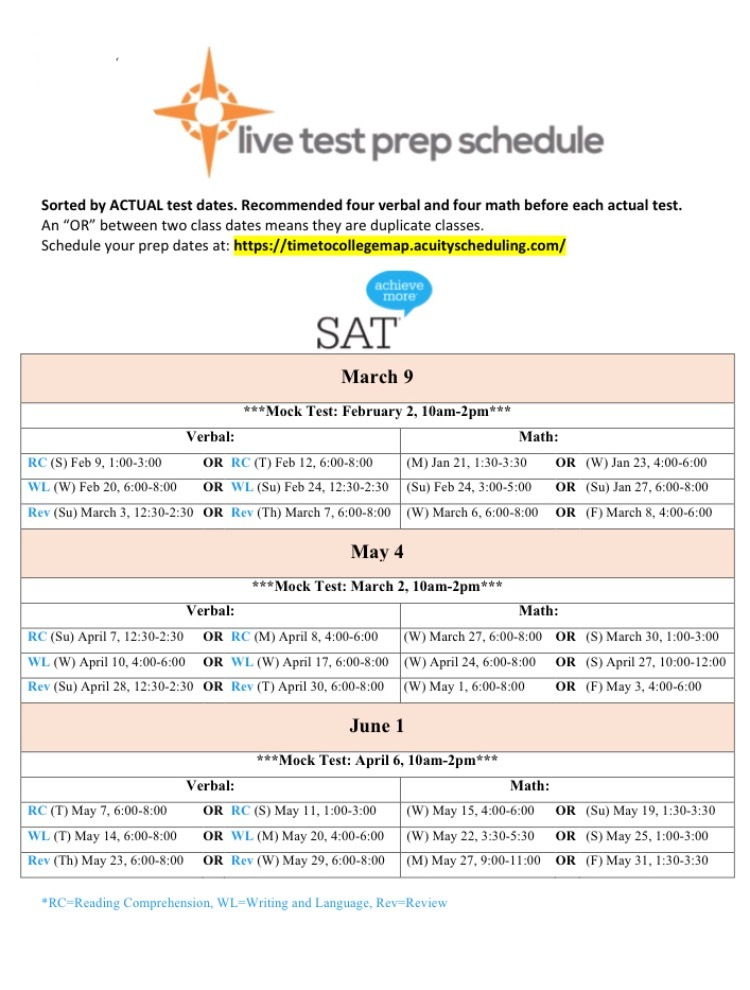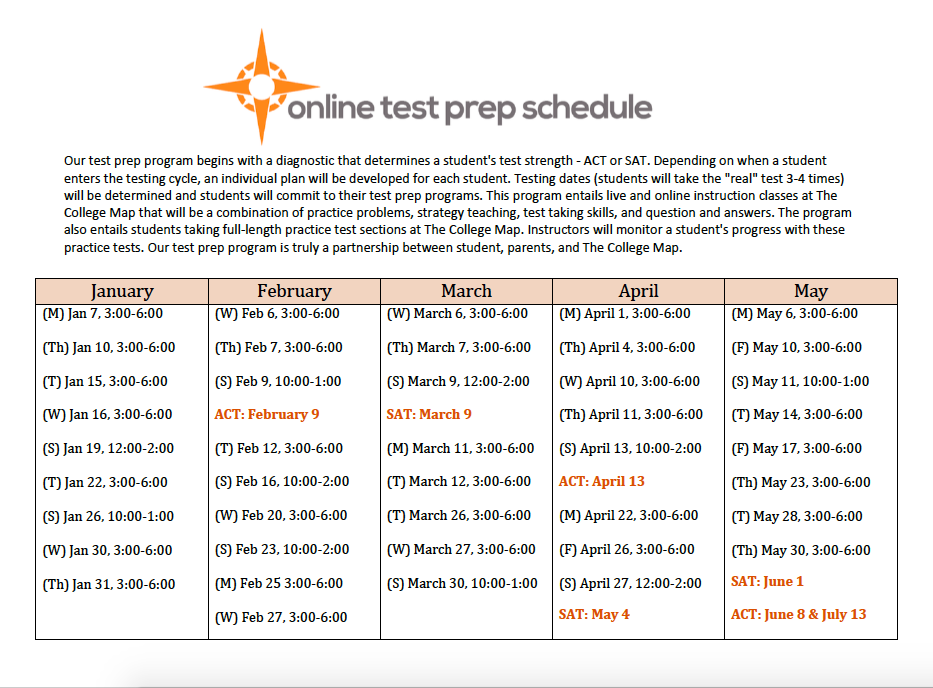table div table+table+table+table+table+table+table+table+table+table+table+table+table+table+table+table+table+table+table+table+table+table+table+table+table+table+table+table+table+table+table div table{width:100%;padding:0}table div table+table+table+table+table+table+table+table+table+table+table+table+table+table+table+table+table+table+table+table+table+table+table+table+table+table+table+table+table+table+table div table img{width:96.23%;padding:0;float:none}table div table+table+table+table+table+table+table+table+table+table+table+table+table+table+table+table+table+table+table+table+table+table+table+table+table+table+table+table+table+table+table div table td{width:100%;padding:0 1.88% 18px}/* styles */## What Is A Mid Year Report?

 /* styles */ What is the mid-year report? Your guidance counselor is required to send a mid-year report to each of the colleges to which you have applied or been accepted into during the early rounds. The basics of the mid-year report are: GPA, class rank (if applicable), and an updated transcript. However, additional information, positive or negative, can be communicated to prospective colleges. The Common App asks counselors to check whether there have been changes in a student’s schedule, disciplinary record, or criminal status. Some institutions will ask similar questions in a more open-ended format. For example, Georgetown University’s mid-year report asks counselors to, “Please comment on any significant additions to or changes in the candidate’s academic, extracurricular, or character record since your previous report and ratings.” - College Transitions
 table div table+table+table+table+table+table+table+table+table+table+table+table+table+table+table+table+table+table+table+table+table+table+table+table+table+table+table+table+table+table+table+table+table+table div table{width:100%;padding:0}table div table+table+table+table+table+table+table+table+table+table+table+table+table+table+table+table+table+table+table+table+table+table+table+table+table+table+table+table+table+table+table+table+table+table div table img{width:96.23%;padding:0;float:none}table div table+table+table+table+table+table+table+table+table+table+table+table+table+table+table+table+table+table+table+table+table+table+table+table+table+table+table+table+table+table+table+table+table+table div table td{width:100%;padding:0 1.88% 18px}/* styles */## DO Something - EASY SCHOLARSHIPS!

 /* styles */ EASY SCHOLARSHIPS Earn easy scholarships through community service Are you between the ages of 13 and 25 looking for scholarship opportunities? Then you’re in the right spot. Here's how to apply for a DoSomething.org college scholarship in 3 easy steps: Sign up for a campaign below Complete the campaign (some take 5 minutes or less!) Upload a photo to show you completed the campaign That’s right: no essays, no GPA requirements, no recommendations, no applications. We've given dozens of scholarships away and are excited to continue supporting students! Read Charlotte's inspiring scholarship story here and Will's incredible scholarship story here. You can enter for multiple scholarships by signing up and completing all of the campaigns listed below. Psst...no need to wait until you're ready for college -- we'll hold on to your award until then! Click here for more information!
 table div table+table+table+table+table+table+table+table+table+table+table+table+table+table+table+table+table+table+table+table+table+table+table+table+table+table+table+table+table+table+table+table+table+table+table+table+table div table{width:100%;padding:0}table div table+table+table+table+table+table+table+table+table+table+table+table+table+table+table+table+table+table+table+table+table+table+table+table+table+table+table+table+table+table+table+table+table+table+table+table+table div table img{width:96.23%;padding:0;float:none}table div table+table+table+table+table+table+table+table+table+table+table+table+table+table+table+table+table+table+table+table+table+table+table+table+table+table+table+table+table+table+table+table+table+table+table+table+table div table td{width:100%;padding:0 1.88% 18px}/* styles */## Athletic Scholarships 101

 /* styles */ Athletic scholarships for college are often misunderstood and assumed to be more available than they are. The process to find these and market your athlete appropriately can be a confusing and overwhelming process. I want to start by providing some general considerations, then talk about the main steps in the process, and finally provide some links to help you get started with finding athletic scholarships and the overall college recruiting process. Read Full Article by Wendy Nelson
 table div table+table+table+table+table+table+table+table+table+table+table+table+table+table+table+table+table+table+table+table+table+table+table+table+table+table+table+table+table+table+table+table+table+table+table+table+table+table+table+table div table{width:100%;padding:0}table div table+table+table+table+table+table+table+table+table+table+table+table+table+table+table+table+table+table+table+table+table+table+table+table+table+table+table+table+table+table+table+table+table+table+table+table+table+table+table+table div table img{width:96.23%;padding:0;float:none}table div table+table+table+table+table+table+table+table+table+table+table+table+table+table+table+table+table+table+table+table+table+table+table+table+table+table+table+table+table+table+table+table+table+table+table+table+table+table+table+table div table td{width:100%;padding:0 1.88% 18px}/* styles */## MEGA list of Scholarships for class of 2019-2020

 /* styles */ Colleges of Distinction You’re about to head off to college, and experience so many exciting things such as taking classes you enjoy, being in an encouraging environment and living on your own. But that also means you’ll need money to support you along the way. Luckily, a lot of companies and organizations want to help you—they offer various grant and scholarship opportunities for students like you. Take advantage of these opportunities and apply to as many as you can to earn up some money. To get you started, we have devised a quick mega list of scholarships you may be eligible for. Full Article Here!
 table div table+table+table+table+table+table+table+table+table+table+table+table+table+table+table+table+table+table+table+table+table+table+table+table+table+table+table+table+table+table+table+table+table+table+table+table+table+table+table+table+table+table+table div table{width:100%;padding:0}table div table+table+table+table+table+table+table+table+table+table+table+table+table+table+table+table+table+table+table+table+table+table+table+table+table+table+table+table+table+table+table+table+table+table+table+table+table+table+table+table+table+table+table div table img{width:96.23%;padding:0;float:none}table div table+table+table+table+table+table+table+table+table+table+table+table+table+table+table+table+table+table+table+table+table+table+table+table+table+table+table+table+table+table+table+table+table+table+table+table+table+table+table+table+table+table+table div table td{width:100%;padding:0 1.88% 18px}/* styles */## When the Answer is NoThere is a scene in the 1998 movie, "You've Got Mail" where the movie's protagonist (played by Meg Ryan) is facing the closing of her quaint bookstore "The Shop Around The Corner" that she inherited from her mother. The movie's antagonist (played by Tom Hanks) has built a mega bookstore resulting in her customers being taken away. Tom Hanks' character justifies his actions by telling Ryan's character that "It isn't personal; it is just business" to which she retorts, "Well, it was personal to ME!"

College rejections just plain hurt. They ARE personal and matter – you wouldn't have applied if you did not care about the outcome. At the core of who we are as people is the need to feel wanted and thus, create a sense of belonging. We simply want to feel "good enough." Sadness and disappointment are expected feelings.

Curiously, at the heart of the word disappointment is "appointment." An appointment is a meeting, an encounter, a type of calling. Your college list is your appointment. There are schools that have accepted you – celebrate them and bask in the knowledge and feeling that you are wanted. Visit those schools again with your eyes and heart open to all of the amazing resources and opportunities available to you.

There is a plan for your life. A rejection is a recalculation to a different place. It is in this different place where you will pursue your academic interests, meet your lifelong friends, and discover what "school spirit" really means. The recalculation may just be a better route to your destination after all!

 table div table+table+table+table+table+table+table+table+table+table+table+table+table+table+table+table+table+table+table+table+table+table+table+table+table+table+table+table+table+table+table+table+table+table+table+table+table+table+table+table+table+table+table+table+table+table div table{width:100%;padding:0}table div table+table+table+table+table+table+table+table+table+table+table+table+table+table+table+table+table+table+table+table+table+table+table+table+table+table+table+table+table+table+table+table+table+table+table+table+table+table+table+table+table+table+table+table+table+table div table img{width:96.23%;padding:0;float:none}table div table+table+table+table+table+table+table+table+table+table+table+table+table+table+table+table+table+table+table+table+table+table+table+table+table+table+table+table+table+table+table+table+table+table+table+table+table+table+table+table+table+table+table+table+table+table div table td{width:100%;padding:0 1.88% 18px}/* styles */## College Visits!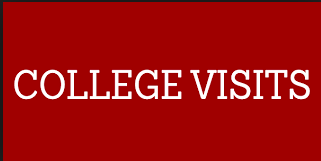College Visits 101

When should I start college visits? Students should begin taking official college visits as early as their freshman year and should not wait until their very busy junior year when it is harder to miss school. In addition, you definitely should not wait to tour schools your senior year.

College visits are important in developing a balanced college list prior to application season. Students need to know whether each school on their application list is a school where they could see themselves.

Will this college be a good fit for me? Do the students on this campus hold my same values? Will I be able to find my people? Does this school offer majors I am interested in? Does this school offer a study abroad program? How large are the classes? Will I be a name or a number? How available are my professors and will my classes be taught by professors?

If you are in high school you should be doing college visits NOW to help answer questions like these.

Please schedule an appointment with your college planning counselor to help prepare for your visits and answer any questions you have.

Don’t delay, schedule your visits today!

 table div table+table+table+table+table+table+table+table+table+table+table+table+table+table+table+table+table+table+table+table+table+table+table+table+table+table+table+table+table+table+table+table+table+table+table+table+table+table+table+table+table+table+table+table+table+table+table+table+table div table{width:100%;padding:0}table div table+table+table+table+table+table+table+table+table+table+table+table+table+table+table+table+table+table+table+table+table+table+table+table+table+table+table+table+table+table+table+table+table+table+table+table+table+table+table+table+table+table+table+table+table+table+table+table+table div table img{width:96.23%;padding:0;float:none}table div table+table+table+table+table+table+table+table+table+table+table+table+table+table+table+table+table+table+table+table+table+table+table+table+table+table+table+table+table+table+table+table+table+table+table+table+table+table+table+table+table+table+table+table+table+table+table+table+table div table td{width:100%;padding:0 1.88% 18px}/* styles */## Bright Futures Information/* styles */ Students must submit a completed Florida Financial Aid Application (FFAA) in order to be considered for State Scholarship & Grant Programs. Seniors, please Create a Student Account. After logging into your account, you may proceed to complete the FFAA. The Bright Futures link can answer all of your questions: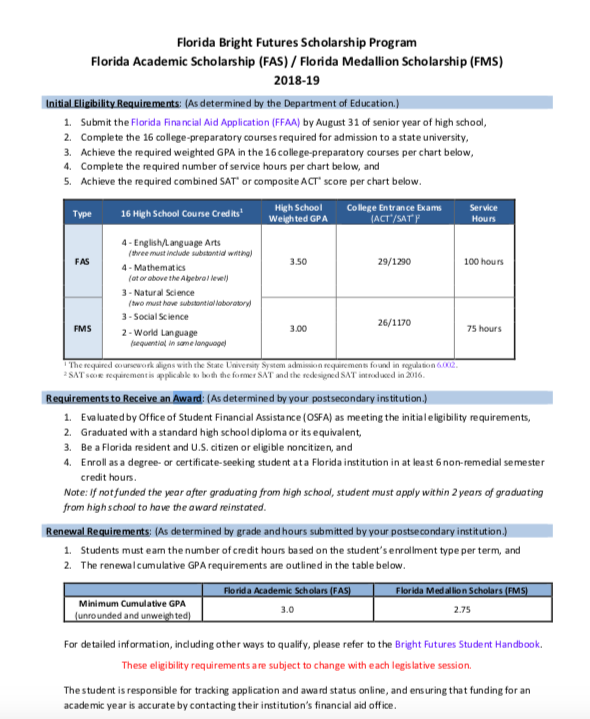table div table+table+table+table+table+table+table+table+table+table+table+table+table+table+table+table+table+table+table+table+table+table+table+table+table+table+table+table+table+table+table+table+table+table+table+table+table+table+table+table+table+table+table+table+table+table+table+table+table+table+table+table+table+table div table{width:100%;padding:0}table div table+table+table+table+table+table+table+table+table+table+table+table+table+table+table+table+table+table+table+table+table+table+table+table+table+table+table+table+table+table+table+table+table+table+table+table+table+table+table+table+table+table+table+table+table+table+table+table+table+table+table+table+table+table div table img{width:96.23%;padding:0;float:none}table div table+table+table+table+table+table+table+table+table+table+table+table+table+table+table+table+table+table+table+table+table+table+table+table+table+table+table+table+table+table+table+table+table+table+table+table+table+table+table+table+table+table+table+table+table+table+table+table+table+table+table+table+table+table div table td{width:100%;padding:0 1.88% 18px}/* styles */## JUNIORS/CLASS OF 2020 SUMMER DATES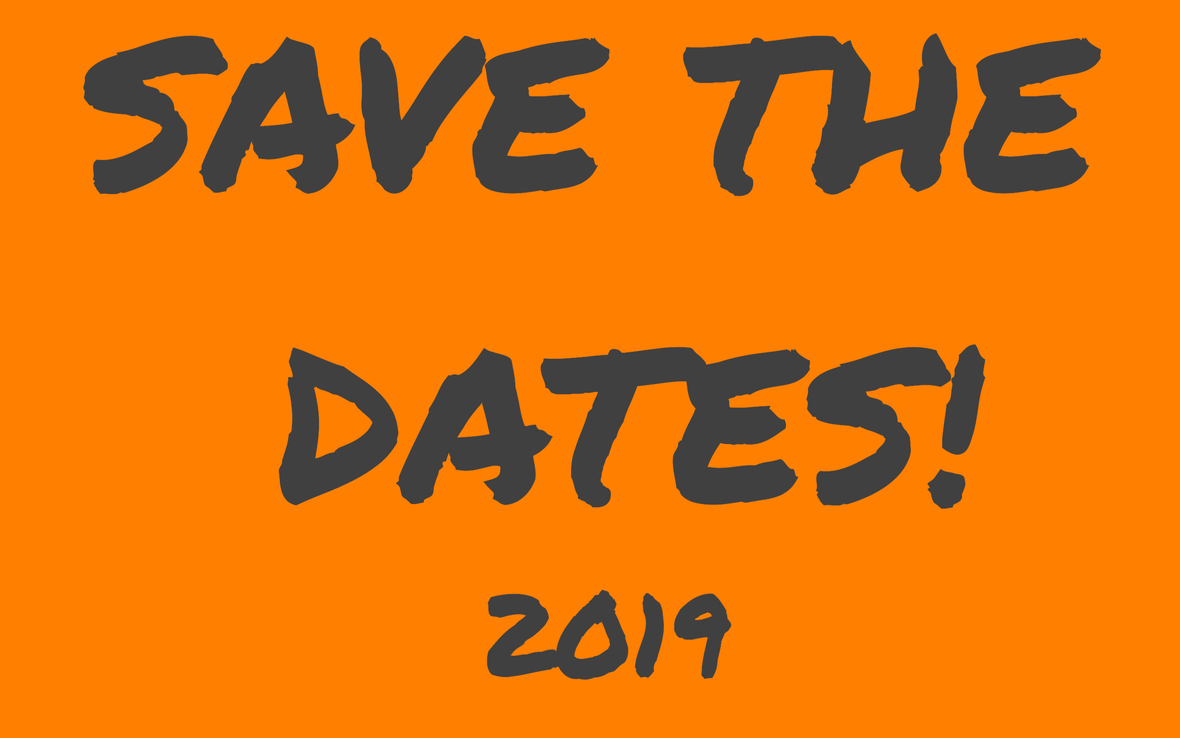/* styles */ ** JUNIORS/CLASS OF 2020 ESSAY WORKSHOP & SENIOR BOOT CAMPS DATES! Writing workshops will be held Saturday, May 18, 2019 and Saturday, June 1, 2019 from 10:00 a.m.until Noon. Students in the class of 2020 must attend one of these two workshops. Please mark your calendars and plan accordingly. Senior Boot Camps for the class of 2020 will be held for two weeks and we also ask that you plan your summer calendar with this in mind and attend all three days of at atleast one of the following weeks: July 23, 24 & 25 or July 30, 31 & August 1st. All dates will be 10:00 a.m. - 6:00 p.m.
 table div table+table+table+table+table+table+table+table+table+table+table+table+table+table+table+table+table+table+table+table+table+table+table+table+table+table+table+table+table+table+table+table+table+table+table+table+table+table+table+table+table+table+table+table+table+table+table+table+table+table+table+table+table+table+table+table+table+table div table{width:100%;padding:0}table div table+table+table+table+table+table+table+table+table+table+table+table+table+table+table+table+table+table+table+table+table+table+table+table+table+table+table+table+table+table+table+table+table+table+table+table+table+table+table+table+table+table+table+table+table+table+table+table+table+table+table+table+table+table+table+table+table+table div table img{width:96.23%;padding:0;float:none}table div table+table+table+table+table+table+table+table+table+table+table+table+table+table+table+table+table+table+table+table+table+table+table+table+table+table+table+table+table+table+table+table+table+table+table+table+table+table+table+table+table+table+table+table+table+table+table+table+table+table+table+table+table+table+table+table+table+table div table td{width:100%;padding:0 1.88% 18px}/* styles */## Scholarships Search Tools

 /* styles */ There are many good scholarship search tools/engines on the internet. Their primary function is to assist you in finding scholarships that match the eligibility criteria you enter for GPA, gender, residency, ethnicity/heritage, religion and area of study, hobbies, interests — some of the most common criteria used. The College Map team recommends the following search engines: Scholarships.com FastWeb Big Future Unigo SallieMae Please make sure to "click" or fill out as much information as possible. Once you've entered your information into the database, the search engine will generate a list of all current scholarships you qualify for. We recommend sorting that list by due date.
 table div table+table+table+table+table+table+table+table+table+table+table+table+table+table+table+table+table+table+table+table+table+table+table+table+table+table+table+table+table+table+table+table+table+table+table+table+table+table+table+table+table+table+table+table+table+table+table+table+table+table+table+table+table+table+table+table+table+table+table+table+table div table{width:100%;padding:0}table div table+table+table+table+table+table+table+table+table+table+table+table+table+table+table+table+table+table+table+table+table+table+table+table+table+table+table+table+table+table+table+table+table+table+table+table+table+table+table+table+table+table+table+table+table+table+table+table+table+table+table+table+table+table+table+table+table+table+table+table+table div table img{width:96.23%;padding:0;float:none}table div table+table+table+table+table+table+table+table+table+table+table+table+table+table+table+table+table+table+table+table+table+table+table+table+table+table+table+table+table+table+table+table+table+table+table+table+table+table+table+table+table+table+table+table+table+table+table+table+table+table+table+table+table+table+table+table+table+table+table+table+table div table td{width:100%;padding:0 1.88% 18px}/* styles */# Website Password

Our private "Your Path" page contains details for upcoming deadlines, events, and important announcements. We encourage you to check it regularly! The password is case-sensitive:
prepare@TCM2018

# Test Prep Schedules

Be sure to register for your spring test prep classes, available on the Your Path page and the Acuity Scheduler. Registration is mandatory; walk-ins may not be able to be accommodated.

# Suggestions?

Is there something you'd like to see in our newsletter? An event you'd like us to host? A friend you'd like to refer? Let us know by simply replying to this message.

 /* styles */ Kind regards, Your Team at The College Map
By |2019-02-18T13:42:01-04:00February 17th, 2019|Your Path|0 Comments